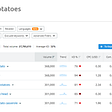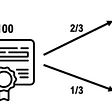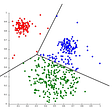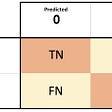# For those who want to get started with data analysis in Python

This article will introduce Jupyter Notebook and Jupyter Lab (collectively called Jupyter), very reliable tools for data analysis in Python.

Jupyter is already in common use in the data science world, but I would like to show its benefits with a demo.

## Assumptions

• Analyze table data, not unstructured data such as images and texts
• Analyze data of several GB or tens of thousands of records, rather than data of several TB or hundreds of millions of records
• Do the exploratory analysis, rather than routine analysis

## What is not written

• How to build Jupyter Notebook and Jupyter Lab environment
• Basic operations of Jupyter Notebook and Jupyter Lab
• How to use pandas data structures and methods

# What a time-consuming process data analysis is!

Exploratory data analysis is time-consuming. I think this is because it requires thousands of trial and error. In conventional development, trial and error often mean fixing a bug in the code or modifying an algorithm. However, there is much more trial and error from the data perspective when it comes to data analysis. You have to look at the data from various angles, verify the quality of the data, and even modify the code when you realize that the data definition you heard from the business department is different…

Therefore, in exploratory data analysis, it is important to be able to do trial and error as quickly as possible.

You also need to report the results of the exploratory data analysis to your boss or clients. Because of the nature of reporting analysis results, the report (PowerPoint, etc.) will contain many tables and graphs, which is an unexpectedly difficult and time-consuming task.

So, it is also important to be able to prepare tables and graphs quickly.

# Two benefits of Jupyter

Time-consuming is the bane of exploratory data analysis but Jupyter can alleviate this bane. For example, it has the following advantages.

• Faster trial and error iteration
- You can get execution results for each row (each cell)
- Variables are saved while Jupyter is running so that you can use them multiple times
• Easy to see the execution results
- Tabular data is easy to read
- Graphs are printed right below the code

I would like to demonstrate these benefits with a demo.

# Demo with rental apartments data

I will use rental apartment data in Chuo-ku, Tokyo that I got from SUUMO(a Japanese rental apartments website) to demonstrate the advantages of Jupyter(*1). I like Jupyter Lab, so I will use it for this demo.

The purpose of the data exploration is to visualize the distribution of rents fee of rental apartments.

First, we need to import pandas and load the data. If a character encoding error occurs due to Japanese or Windows characters, pass encoding=’CP932' as an argument.

`# Load the libraryimport pandas as pd# Read in the dataapart = pd.read_csv('apartments_20210410_chuo.csv')`

Once the data has been read, use the head() method to display and check the data. This head() method is so good that you can see the tabular data very easily and clearly. In my opinion, it is possible to use this table’s screenshot for reports. (Of course, it depends on who you are reporting to. If you are working with an external client, it is better to export to a CSV file and use a PowerPoint table.)

`# Display the dataapart.head()`

The default output is 5 lines, but you can change the output lines passing a number as an argument. In my usage, I use 5 lines (default) when I want to see the columns and values of the data, 1 line when I want to save the data to see later, and 100 lines when I want to see the data itself.

The purpose of this demo is to visualize the rent. The rent is in the form of “10 万円” which contains kanji so we need to omit these characters and convert them into an int type number.

We will combine the lambda expression with the map function to fix the rent column. One of the advantages of Jupyter is that you can iterate like this, thinking and executing processes on the fly. (This may be a good point about the interactive environment rather than Jupyter…)

`# Erase '円'# If there is '万', remove it and multiply by 10000apart[‘rent_yen’] = list(map(lambda x: x.replace(‘円’, ‘’), apart.rent))apart[‘rent_yen’] = list(map(lambda x: float(x.replace(‘万’, ‘’))*10000 if ‘万’ in x else x, apart.rent_yen))apart[‘rent_yen’] = apart[‘rent_yen’].astype(‘int’)`

There is a function called apply() in pandas that can do the same thing as map(), but I recommend using map() for its speed. However, map() can only process one column of the DataFrame at a time, so if you need to process values from multiple columns in one row at the same time, use apply().

By the way, when you rent an apartment in Japan, you usually sign a two-year contract. condominium fees and gratuity are also required. To calculate the whole cost more accurately, let’s try to calculate the cost over two years. Specifically, we will calculate the sum of two years of rent (24 months), plus two years of condominium fees (24 months), plus the gratuity.

`# Erase ‘円’# If there is ‘万’, remove it and multiply by 10000apart[‘condo_fee_yen’] = list(map(lambda x: x.replace(‘円’, ‘’), apart.condo_fee))apart[‘condo_fee_yen’] = list(map(lambda x: float(x.replace(‘万’, ‘’))*10000 if ‘万’ in x else x, apart.condo_fee_yen))apart[‘condo_fee_yen’] = apart[‘condo_fee_yen’].astype(‘int’)`

We will convert the condominium fee into yen the same as rent.

But when we applied the same function, we get an error. It seems that it could not be converted to a numeric type because there was a hyphen.

We check the data and we will see that hyphen indicates that the condominium fee is free.

Even if errors occur, Jupyter itself is still running. Thus the variables and libraries that have been calculated and loaded are still alive, so we can try again. This is another advantage of Jupyter.

We’ll create a function to handle hyphens, but it’s a bit too complicated to write it as a lambda function so we’ll write it as a method.

`def extract_jpy(x):  “””  — Erase ‘円’  — hyphen is replaced by 0  — If there is ‘万’, remove it and multiply by 10000  — convert into integer  “””  x = x.replace(‘円’, ‘’)  x = x.replace(‘-’, ‘0’)  if ‘万’ in x:    x = x.replace(‘万’, ‘’)    x = float(x)*10000  return int(x)apart[‘condo_fee_yen’] = list(map(extract_jpy, apart.condo_fee))`

It looks like we have successfully converted the condominium fee into a number.

We can now do the same for the gratuity.

`apart[‘gratuity_yen’] = list(map(extract_jpy, apart.gratuity))`

Since we are doing the same processing here as for the condominium costs, we can copy the cells and use them. Jupyter has some useful shortcut keys that can be used for quick operations. In particular, I often use c: copy cell, x: cut cell, v: paste cell, z: undo cell operation, a: add new cell above, b: add new cell below. I also recommend using ESC: switch to cell operation mode and Enter: switch to code input mode, as they will accelerate your work.

We can see the three columns we have created have been handled well.

`apart.head()`

It looks like “rent_yen”, “condo_fee_yen”, and “gratuity_yen” are all well extracted as numerical values.

Now, let’s calculate the whole cost for two years using the pandas apply function.

`apart[‘cost_2years’] = apart.apply(lambda x: (x.rent_yen + x.condo_fee_yen)*24 + x.gratuity_yen, axis=1)apart.head()`

It looks like we have successfully calculated the whole cost over two years. We are now ready to visualize the data.

Now we will visualize the data. We will use plotly. This is my favorite library because of its ease of use and beautiful visualization. In particular, the appearance is great so that it can be used for PowerPoint as is. (Unlike seaborn/matplotlib, Japanese is not garbled by default, which is also nice.)

`# Load the libraryimport plotly.express as px# Visualizefig = px.histogram(apart, x='cost_2years')fig.show()`

The histogram shows a wide distribution, from 200k to 23M. 20M JPY apartments are too expensive to live for me, so we’ll filter the threshold to 10M, which covers most of the data.

`fig = px.histogram(apart.query(‘cost_2years <= 10000000’), x=’cost_2years’)fig.show()`

We can see that there are several mountains in this graph. The distribution might be different depending on the room layout, so let’s try to visualize it by color-coding according to the layout.

`fig = px.histogram(apart.query(‘cost_2years <= 10000000’), x=’cost_2years’, color=’layout’,barmode=’overlay’)fig.show()`

We can see that the distribution differs depending on the room layout. Now let’s compare the distribution of 1K and 1LDK. Since most of the data is up to 7M JPY, we will filter by 7M.

`fig = px.histogram(apart.query(‘cost_2years <= 7000000 and (layout == “1K” or layout == “1LDK”)’), x=’cost_2years’, color=’layout’,barmode=’overlay’)fig.show()`

We can now visualize that the distribution is neatly divided into two mountains. This is the end of the analysis in this demo, but there are many things to explore, such as what contributes to the price distribution besides the room layout.

In this way, Jupyter is efficient when you look at data for the first time, and you don’t know what kind of data, what kind of data type, what kind of data format, and what kind of distribution, or the work that needs to be done comes up while exploring data.

# Appendix: Issues with Jupyter and how to solve them

Finally, I’d like to list some of my concerns about using Jupyter and how to handle them. The increase of technical debt is a problem not only for Jupyter, but also for machine learning systems, and I think there is still room for improvement.

• Appearance
> In JupyterLab, you can choose a dark theme by default.
• Code Completion
> Use a library for completion (such as jupyterlab-lsp)
• Increasing technical debt
> Use jupytext to generate .py files and version them with git
> Cut code into py files as needed and use them as methods
> Write documentation
> Write test code

*1: Data collection from websites for data analysis doesn’t violate any laws in Japan unless it relates to personal information or it putting a high workload on the servers.

--

--

--

## More from Ryo Koyajima / 小矢島 諒

Love podcasts or audiobooks? Learn on the go with our new app.

## How to do SEMRush Style Keyword Grouping with Python## To Catch a Cambrioleur: Policing France’s Open Data## Exploratory Data Analysis(EDA) — 30%-40% of Data Science Project## Types of Observational Studies in Statistics## Money for Nothing: An Arbitrage Paradox## K-Means Clustering Algorithm## A python function to get all the possible stats from a confusion matrix## Central Limit Theorem: Let’s learn with an example using Python## An Introduction To Statistics With Python — Part 2